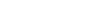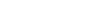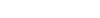# DERIVATION OF UNIT HYDROGRAPHSReading time: 1 minute

DERIVATION OF UNIT HYDROGRAPHS

1. A number of isolated storm hydrographs caused by short spells of rainfall excess, each of approximately the same duration (0.9 to 1.1D h) are selected from a study of continuously gauged runoff of the stream

2. For each of these surface runoff hydrographs, the base flow is separated

3. The area under DRH is evaluated and the volume of direct runoff obtained is divided by the catchment area to obtain the depth of ER

4. The ordinates of the various DRHs are divided by the respective ER values to obtain the ordinates of the unit hydrograph

Flood hydrographs used in the analysis should be selected so as to meet the following desirable features with respect to the storms responsible for them:

1. The storms should be isolated storms occurring individually

2. The rainfall should be fairly uniform during the duration and should cover the entire catchment area

3. The duration of rainfall should be 1/5 to 1/3 of the basin lag

4. The rainfall excess of the selected storm should be high (A range of ER values of 1.0 to 4.0 cm is preferred)

• A number of unit hydrographs of a given duration are derived as mentioned above and then plotted

• Because of spatial and temporal variations in rainfall and due to deviations of the storms from the assumptions in the unit hydrograph theory, the various unit hydrographs developed will not be exactly identical

• In general, the mean of these curves is adopted as the unit hydrograph of the given duration for the catchment

• The average of the peak flows and the time to peaks are computed first

• Then a mean curve of best fit (by eye judgment) is drawn through the averaged peak to close on an averaged base length

• The volume of the DRH is determined and any departure from unity is corrected by adjusting the peak value

Note – It is customary to draw the averaged ERH of unit depth in the plot of the unit hydrograph to indicate the type and duration of rainfall creating the unit hydrograph.Fig: Derivation of an average unit hydrograph

• It is assumed that the rainfall excess occurs uniformly over the catchment during the duration D hours of a unit hydrograph

• An ideal duration for a unit hydrograph is one in which small fluctuations in rainfall intensity does not have any significant effect on the runoff

• The duration of the unit hydrograph should not exceed 1/5 to 1/3 of the basin lag

• In general, for catchments larger than 250sq.km., 6 hour duration is satisfactory.

#### Unit Hydrograph from a Complex Storm

• When suitable simple isolated storms are not available, data from complex storms of long duration will have to be used to derive the unit hydrograph

• The problem is to decompose a measured composite flood hydrograph into its component DRHs and base flow

• A common unit hydrograph of appropriate duration is assumed to exist

• This is the inverse problem of derivation of the flood hydrograph

• Consider a rainfall excess made up of three consecutive durations of D hours and ER values of.

• After base flow separation of the resulting composite flood hydrograph, a composite DRH is obtained. Let the ordinates of the composite DRH be drawn at a time interval of D hours.

• At various time intervals 1D, 2D, 3D, ……. from the start of the ERH let the ordinates of unit hydrograph beand the ordinates of the composite DRH beFigure: Unit hydrograph from a complex storm• The values ofcan be determined from the above

• Disadvantage of this method – Errors propagate and increase as computation proceeds